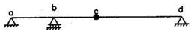# A very wide rectangular channel is designed to carry a discharge of 5 m3/s per meter width. The design is based on the Manning's equation with the roughness coefficient obtained from the grain size using Strickler's equation and results in a normal depth of 1.0 m. By mistake, however, the engineer used the grain diameter in mm in the Strickler's equation instead of in meter. What should be the correct normal depth

1.  0.32 m

2.  0.50 m

3.  2.00 m

4.  3.20 m

4

2.00 m

Explanation :
No Explanation available for this question

# The flow of glycerin (kinematic viscosity v = 5x10-4 m2/s) in an open channel is to be modeled in a laboratory flume using water (v = 10-6 m2/s) as the flowing fluid. If both gravity and viscosity are important, what should be the length scale (i.e. ratio of prototype to model dimensions) for maintaining dynamic similarity

1.  1

2.  22

3.  63

4.  500

4

63

Explanation :
No Explanation available for this question

# Considering beam as axially rigid, the degree of freedom of a plane frame shown below is

1.  9

2.  8

3.  7

4.  6

4

6

Explanation :
No Explanation available for this question

# For a linear elastic frame, if stiffness matrix is doubled, the existing stiffness matrix, the deflection of the  resulting frame will be

1.   twice the existing value

2.   half the existing value

3.   the same as existing value

4.   indeterminate value

4

the same as existing value

Explanation :
No Explanation available for this question

# A clayey soil has a maximum dry density of 16 kN/m3 and optimum moisture content of 12%. A contractor during the construction of core of an earth dam obtained the dry density 15.2 kN/m3 and water content 11%. This construction is acceptable because.

1.  the density is less than the maximum dry density and water content is on dry side of optimum.

2.  the compaction density is very low and water content is less than 12%.

3.  the compaction is done on the dry side of the optimum.

4.  both the dry density and water content of the compacted soil are within the desirable limits

4

both the dry density and water content of the compacted soil are within the desirable limits

Explanation :
No Explanation available for this question

# An inert tracer is injected continuously from a point in an unsteady flow field. The locus of locations of all the tracer particles at an instance of time represents

1.   Streamline

2.   Pathline

3.   Steamtube

4.   Streakline

4

Streakline

Explanation :
No Explanation available for this question

# Consider the following two statements I.The maximum number of linearly independent column vectors of a matrix A is called the rank of A II. If A is an n x n square matrix, it will be nonsingular if rank A = n. With reference to the above statements, which of the following applies

1.  Both the statements are false

2.  Both the statements are true

3.  I is true but II is false

4.  I is false but II is true

4

Both the statements are true

Explanation :
No Explanation available for this question

# A two span beam with an internal hinge is shown below The conjugate beam corresponding to this beam is

1.

2.

3.

4.

4Explanation :
No Explanation available for this question

# A frame ABCD is supported by a roller at A and is on a hinge at C as shown below The reaction at the roller end A is given by

1.  P

2.  2 P

3.  P/2

4.  Zero

4

Zero

Explanation :
No Explanation available for this question

1.  50%

2.   66%

3.  100%

4.  33%

4